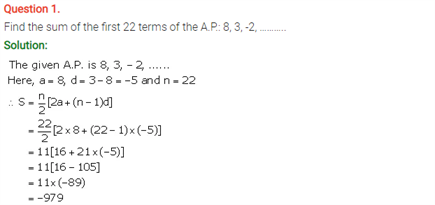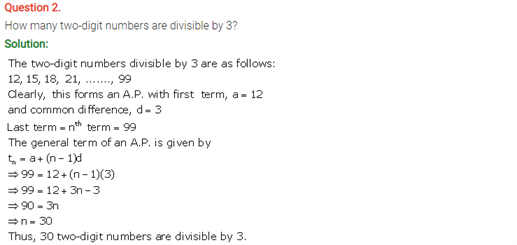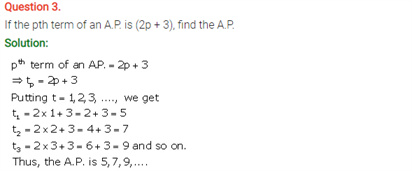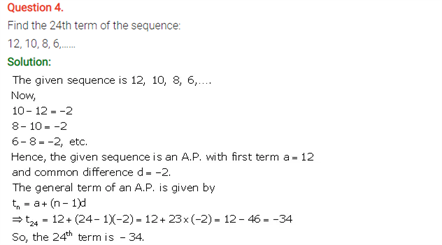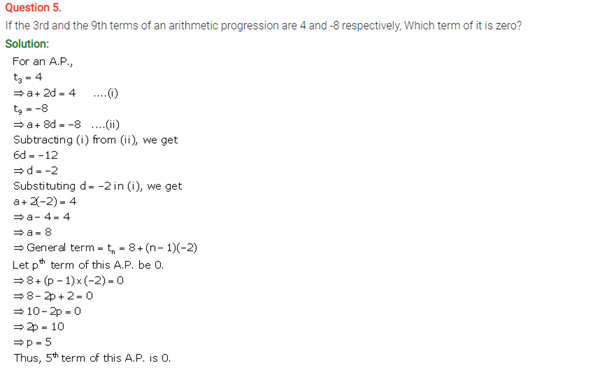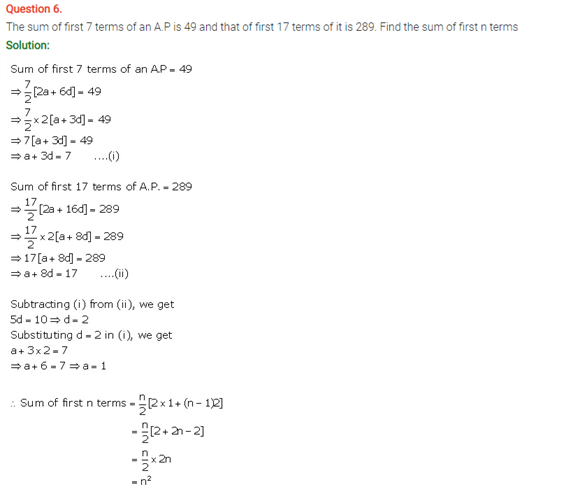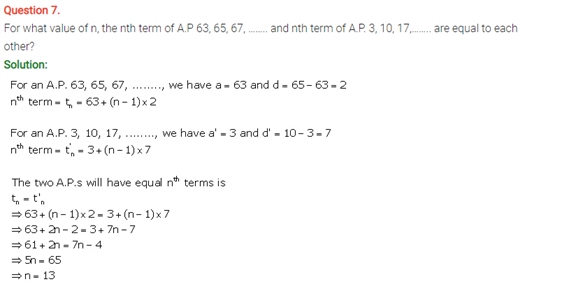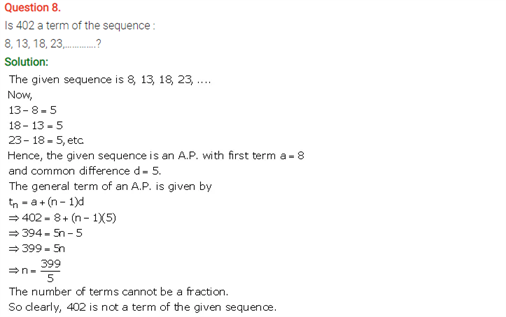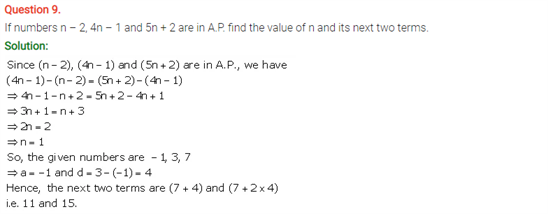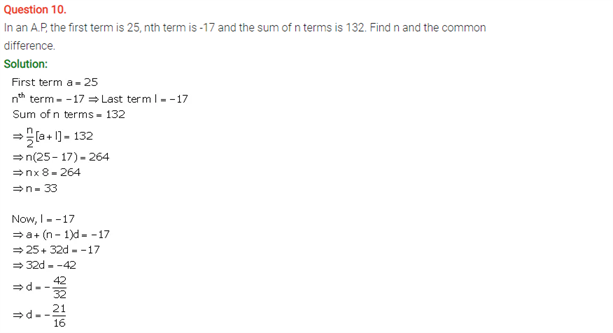# Selina Concise Maths Solution for ICSE Class 10 Chapter 10 Arithmetic Progression

## Selina Class 10 Maths Solutions for Chapter 10

ICSE Solutions For Class 10 Maths is prepared by our experts which brings you the excitement of solving all the important questions of this chapter. An Arithmetic progression is referred to the sequence of numbers in which the difference between two subsequent numbers is a constant. For example: the sequence 20, 10, 0, -10, -20, -30 is said to be in arithmetic progression with common difference as -10. Similarly, you could find various concept on A.P and solving the solutions will enable a better grasp.

You will find the ICSE Selina Solutions for Class 10 Maths Chapter 10 Arithmetic Progression below: